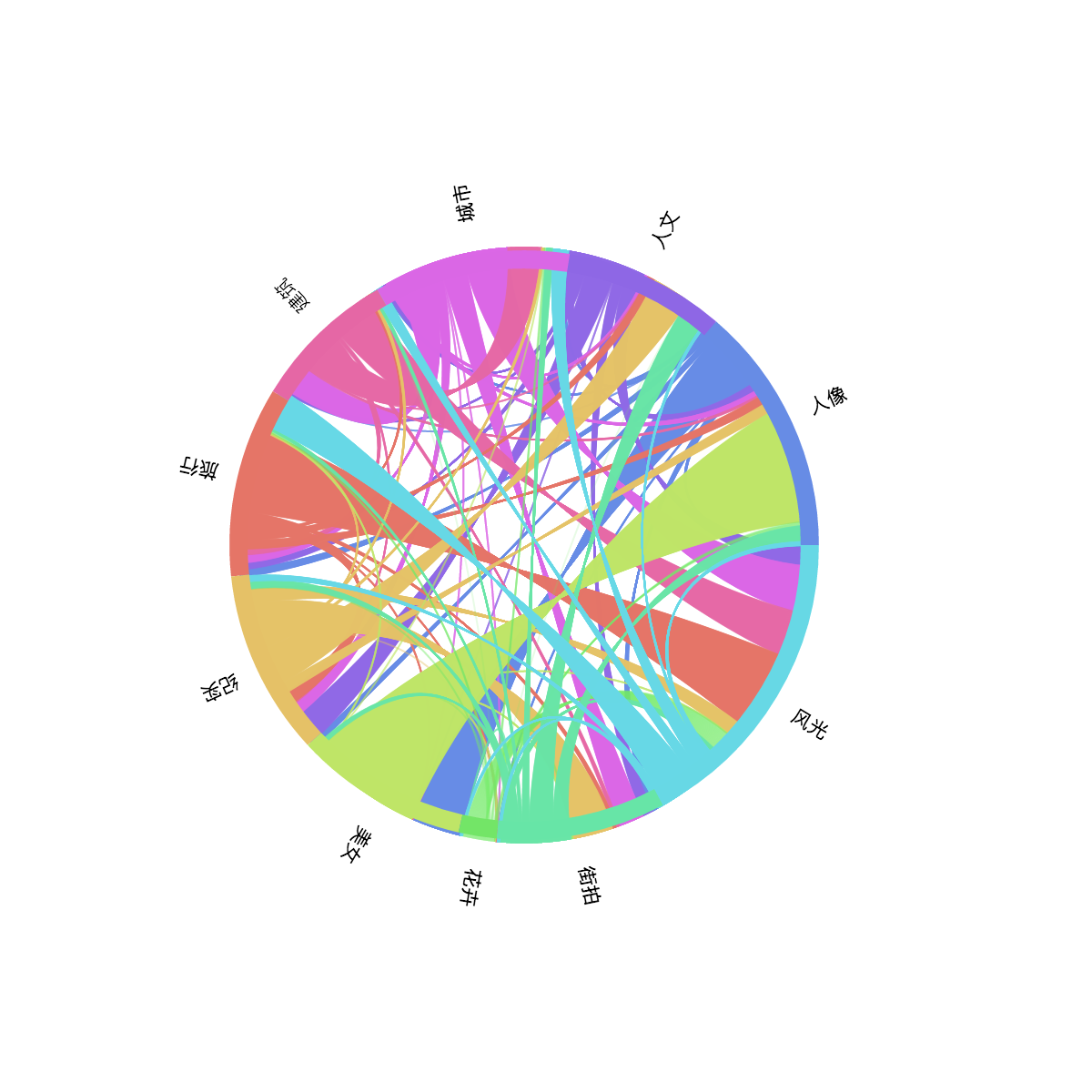# 用神经网络模型给你的照片打分（Part I）

## 问题

1. 最流行的相机型号排名；
2. 不同型号相机拍摄的照片所获得的平均得分；
3. 不同标签的照片最常使用的相机型号分布；
4. 照片标签之间的相互关联关系。

```import pandas as pd
cameraDF = pd.read_pickle("cameraInfo.pkl")
cameraDF.head()
```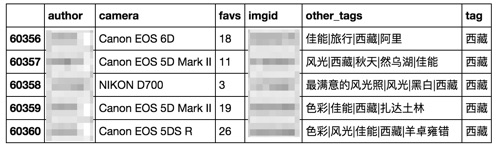```from bokeh.plotting import figure, show
from bokeh.io import output_notebook
from bokeh.models import HoverTool
output_notebook()
```

### 1. 最流行的相机型号 Top10

```cameraCount = cameraDF.camera.value_counts().head(10).to_frame('Count')
cameraCount
```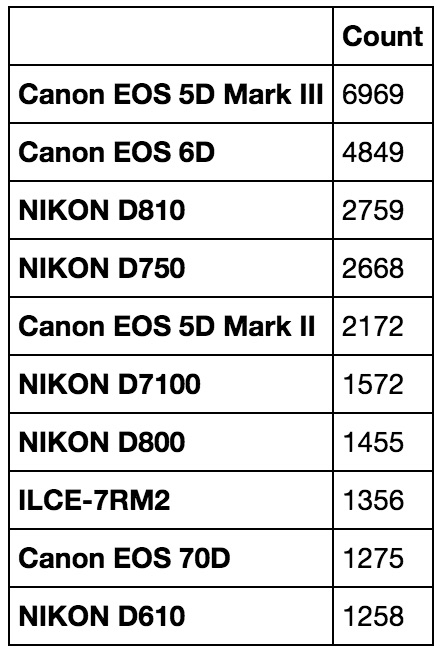```from bokeh.charts import Bar
from bokeh.charts.attributes import cat
bar = Bar(cameraCount, label=cat(sort=False), values='Count', title="相机型号 Top10", legend=None)
bar.xaxis.axis_label = None
bar.yaxis.axis_label = None
bar.title.align = 'center'
bar.width = 900
bar.tools = []
show(bar)
```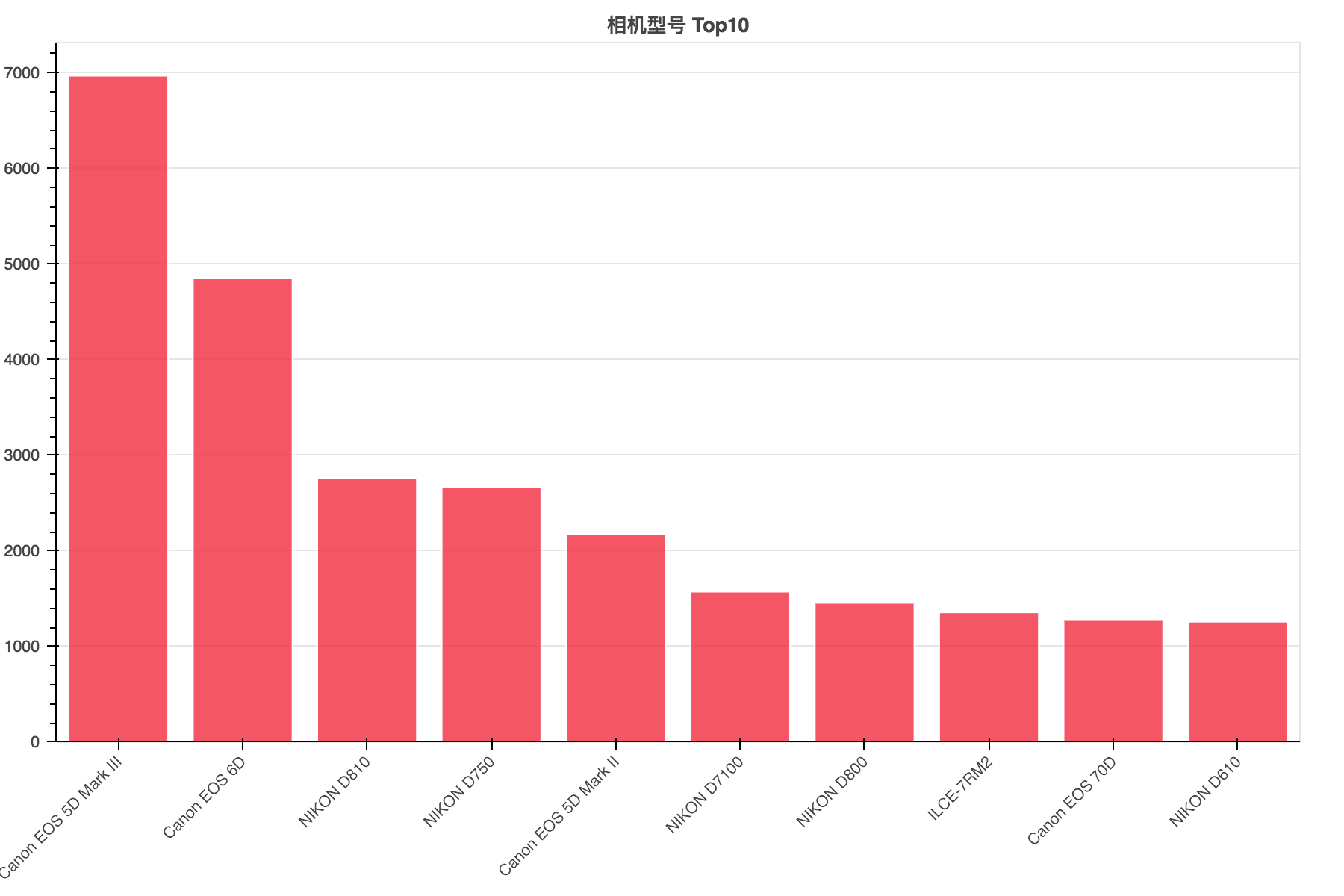### 2. 相机型号所拍照片平均得分

```cameraScores = cameraDF.groupby('camera') \
.filter(lambda x: len(x) > 500) \
.groupby('camera')['favs'].mean() \
.to_frame('M')
cameraScores.head()
```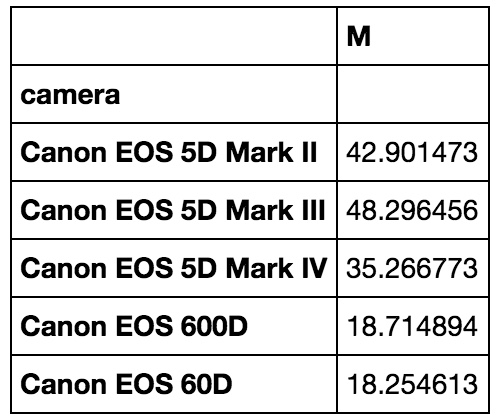```from bokeh.charts.attributes import cat
box = Bar(cameraScores.sort_values('M', ascending=False),
values='M',
label=cat(sort=False),
legend=None,
title='平均得分')
box.title.align = 'center'
show(box)
```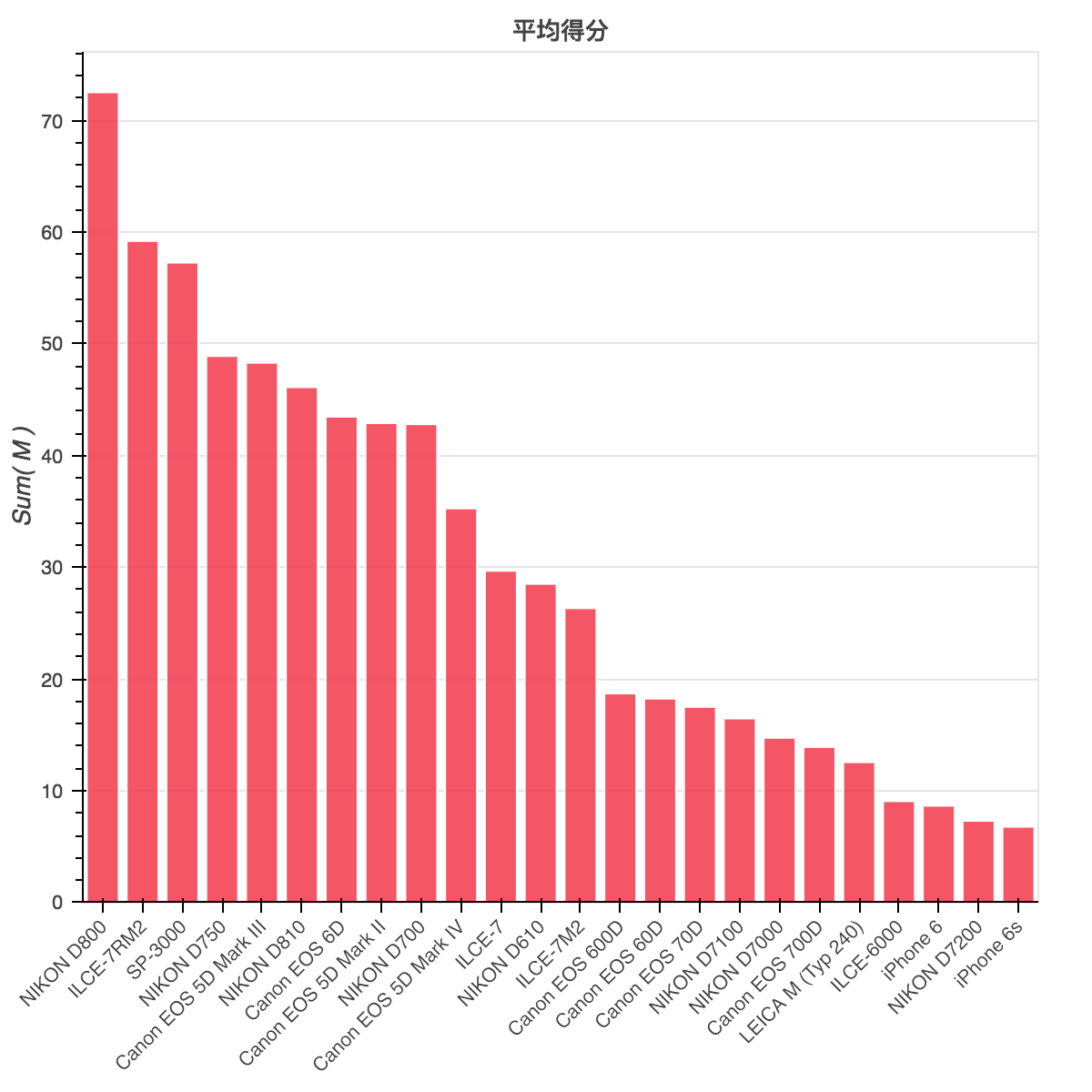### 3. 不同标签（类型）照片最常用设备

```TOI = cameraDF.tag.unique()[:10]
tagOfInterest = cameraDF[cameraDF.tag.apply(lambda x: x in TOI)]

tagOfInterest = tagOfInterest.groupby(['tag', 'camera'])\
.count().reset_index()\
.sort_values(['tag', 'author'], ascending=False)\
.groupby('tag').head(3)
tagOfInterest['total'] = tagOfInterest.author
```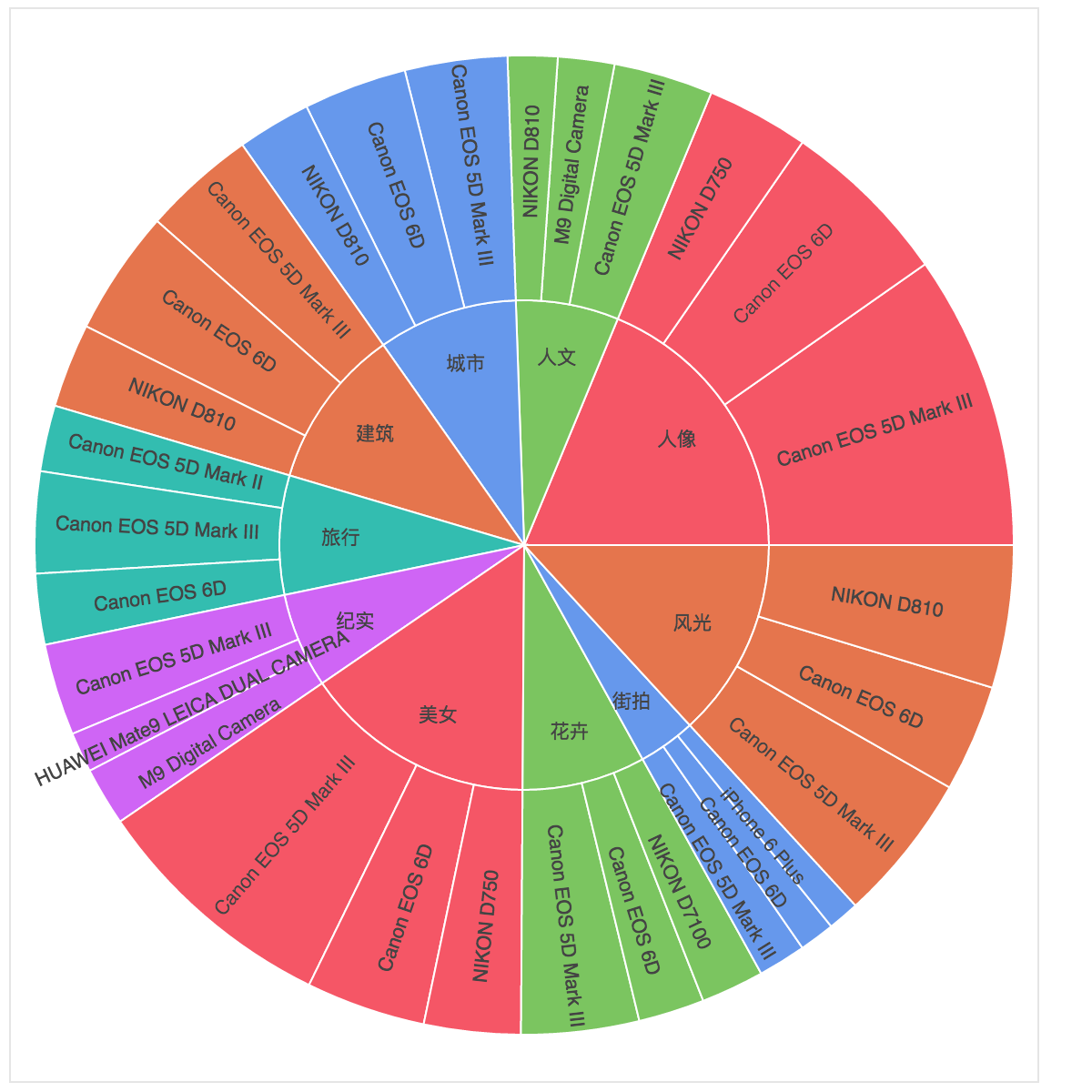### 3. 照片标签之间的关联

```TagRelationship = []
for i in range(len(cameraDF)):
tag = cameraDF.loc[i, 'tag']
other_tags = cameraDF.loc[i, 'other_tags']
if tag in TOI:
for o_t in other_tags.split("|"):
if o_t != tag and o_t in TOI:
TagRelationship.append({'main_tag': tag, 'rel_tag': o_t, 'value': 1})

tagRelSum = TagRelationshipDF.groupby(['main_tag', 'rel_tag'])['value'].sum() \
.to_frame().reset_index()

from bokeh.charts import Chord
chd = Chord(tagRelSum, source='main_tag', target='rel_tag', value='value')
show(chd)
```